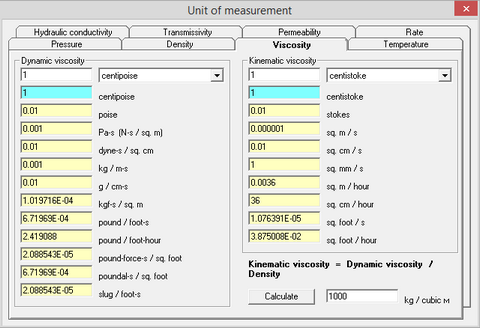﻿ Help > Unit converter (Part 1) > Viscosity

# Viscosity

Navigation:  Help > Unit converter (Part 1) >

# Viscosity

The viscosity of a fluid is a measure of its resistance to deformation at a given rate.

The term "viscosity" is differentiated into dynamic viscosity (units: poise, Pa·s) and kinematic viscosity (units: stokes, m2/s). Kinematic viscosity is determined as the ratio of dynamic viscosity to fluid density. The term and definition originate from classical methods of viscosity measurements, such us measuring time during which a defined volume of fluid discharges under gravity force through a hole of defined size.

To convert dynamic viscosity into kinematic viscosity, the button "Calculate" on the bottom of the dialog window below can be used.Tab "Viscosity" of the dialog window "Unit converter (part 1)"

 Poise The poise is the unit of dynamic viscosity (absolute viscosity) in the CGS system of units. It is named after Jean Léonard Marie Poiseuille. 1 poise = 1 g / (cm·s) = 0.1 N·s/m2 Water at temperature 25 °C has viscosity of 0.008937 poise Pascal-second (Pa·s), Pascal-second is the unit of dynamic viscosity (absolute viscosity) in the SI system of units. 1 Pa·s = 1 N·s/m2 = 10 poise Stokes (St) The stokes is the CGS unit for kinematic viscosity. The unit is named after Sir George Gabriel Stokes. 1 St = cm2/s = 10-4 m2/s. In practice, often 1/100 unit of stokes is used - centistokes (cSt). 1 cSt = 1 mm2/s Square meter per second (m2/s) This is the unit of kinematic viscosity in the SI system of units. 1 m2/s = 10000 stokes